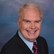# Math Solutions: Poundage CalculationsBy Daniel Theobald, "Wastewater Dan"

Water Online’s “Math Solutions,” presented by wastewater consultant and trainer Dan Theobald (“Wastewater Dan”), instructs operators on poundage calculations.

Plant operations or certification exams may require operators to figure out gallons/flow, concentration of parameters, or other calculations related to poundage. Depending on your required math solution, calculations and unit of expression require explicit formulas.

Some common poundage calculations are accessible below:

Calculate Pounds

Calculate pounds of lime in slurry tank (using concentration as %):

Calculate pounds of solids in sludge tank (using concentration as milligrams per liter [mg/L]):

Calculate pounds of biochemical oxygen demand (BOD)/day (using flow):

Calculations Using Pounds

Calculate gallons in aeration tank using pounds of solids:

Calculate BOD mg/L per day using flow and pounds:

Complete playlist of Wastewater Dan’s Math Solutions

This is the Poundage Calculations presentation in my series of “Math Solutions.” If you have specific wastewater math queries, please submit a question.